## A hypothetical Planet X of mass 7.5 ✕ 1023 kg rotates on its axis once every 5 days. Hint (a) Find the distance from the center of Planet X

Question

A hypothetical Planet X of mass 7.5 ✕ 1023 kg rotates on its axis once every 5 days. Hint (a) Find the distance from the center of Planet X (in m) at which a satellite would remain in one spot above the planet’s surface. m (b) Find the speed of the satellite (in m/s). m/s

in progress 0
3 months 2021-08-06T09:55:19+00:00 1 Answers 14 views 0

(A) The distance of the center of Planet X is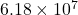m

(B) The speed of the satellite is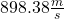Explanation:

Given:

Mass of the planet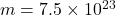kg

Time period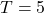days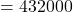sec

(A)

From kepler’s third law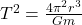Where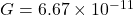Now for finding the distance of planet X,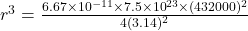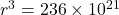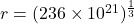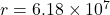m

(B)

Speed of the satellite is given by,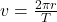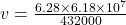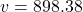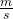Therefore, the speed of planet is